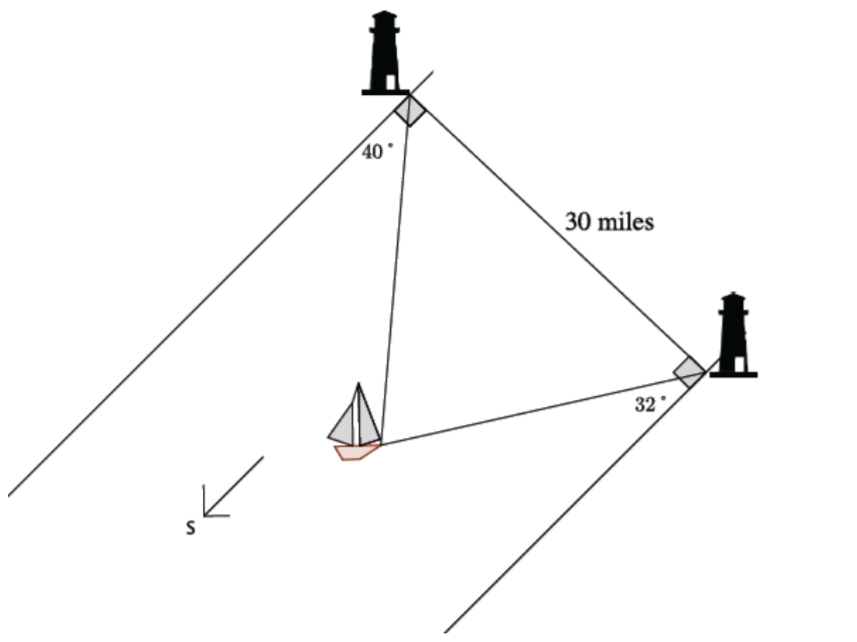# Right Triangles and Trigonometry

## Objective

Use side and angle relationships in right and non-right triangles to solve application problems.

## Common Core Standards

### Core Standards

?

• G.SRT.D.11 — Understand and apply the Law of Sines and the Law of Cosines to find unknown measurements in right and non-right triangles (e.g., surveying problems, resultant forces).

## Criteria for Success

?

1. Model a contextual situation in a diagram, labeling all relevant measures and annotating the diagram with important information.
2. Identify the most efficient and appropriate theorem to find the missing information in a contextual problem.
3. Solve application problems efficiently and interpret the solution in the context of the problem.
4. Verify solution method and justify reasoning.

## Anchor Problems

?

### Problem 1

A surveyor needs to determine the distance between two points, $A$ and $B$, that lie on opposite banks of a river. A point $C$ is chosen ${160}$ meters from point $A$, on the same side of the river as $A$. The measures of $\angle BAC$ and $\angle ACB$ are 41° and 55°, respectively. Approximate the distance from $A$ to $B$ to the nearest meter.

#### References

EngageNY Mathematics Geometry > Module 2 > Topic E > Lesson 32Example 1

Geometry > Module 2 > Topic E > Lesson 32 of the New York State Common Core Mathematics Curriculum from EngageNY and Great Minds. © 2015 Great Minds. Licensed by EngageNY of the New York State Education Department under the CC BY-NC-SA 3.0 US license. Accessed Dec. 2, 2016, 5:15 p.m..

### Problem 2

Two lighthouses are 30 miles apart on each side of the shorelines running north and south, as shown. Each lighthouse keeper spots a boat in the distance. One lighthouse keeper notes the location of the boat as 40° east of south, and the other lighthouse keeper marks the boat as 32° west of south. What is the distance from the boat to each of the lighthouses at the time it was spotted? Round your answers to the nearest mile.#### References

EngageNY Mathematics Geometry > Module 2 > Topic E > Lesson 33Example 3

Geometry > Module 2 > Topic E > Lesson 33 of the New York State Common Core Mathematics Curriculum from EngageNY and Great Minds. © 2015 Great Minds. Licensed by EngageNY of the New York State Education Department under the CC BY-NC-SA 3.0 US license. Accessed Dec. 2, 2016, 5:15 p.m..

## Problem Set

?

The following resources include problems and activities aligned to the objective of the lesson that can be used to create your own problem set.

• Include problems where students choose the most efficient and appropriate theorem to find the missing information in a contextual problem.